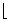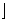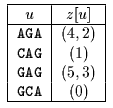Name
• String
• Substring
• Search
Edit

The preprocessing phase of the Alpha Skip Search algorithm consists in building a trie T(x) of all the factors of the length=logm occurring in the word x. The leaves of T(x) represent all the factors of lengthof x. There is then one bucket for each leaf of T(x) in which is stored the list of positions where the factor, associated to the leaf, occurs in x.

The worst case time of this preprocessing phase is linear if the alphabet size is considered to be a constant.

The searching phase consists in looking into the buckets of the text factors y[j .. j+-1] for all j = k.(m-+1)-1 with the integer k in the interval y[1,(n-) / m].

The worst case time complexity of the searching phase is quadratic but the expected number of text character comparisons is O(log(m).(n / (m-log(m)))).

Main features:

improvement of the Skip Search algorithm;

uses buckets of positions for each factor of length log(m) of the pattern;

preprocessing phase in O(m) time and space complexity;

searching phase in O(mn) time complexity;

O(log(m).(n / (m-log(m)))) expected text character comparisons.

Example:

Preprocessing phaseZ table used by Alpha Skip Search algorithm.

Searching phase

 G C A T C G C A G A G A G T A T A C A G T A C G 1 2 3 G C A T C G C A G A G A G T A T A C A G T A C G - - - 4 5 6 7 8 G C A G A G A G

Shift by: 6

 G C A T C G C A G A G A G T A T A C A G T A C G 1 2 3

Shift by: 6

 G C A T C G C A G A G A G T A T A C A G T A C G 1 2 3 G C A T C G C A G A G A G T A T A C A G T A C G 1 - - - G C A G A G A G

The Alpha Skip Search algorithm performs 18 character comparisons on the example.

C